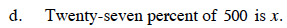Home > CCA2 > Chapter 11 > Lesson 11.1.3 > Problem11-35

11-35.
1. Solve each ratio problem below for x. Homework Help ✎

1. Forty-two percent of x is 112.

2. Forty-two is x percent of 112.

3. Twenty-seven is x percent of 100.

4. Twenty-seven percent of 500 is x.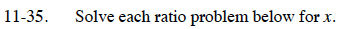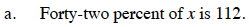0.42x = 112

x ≈ 266.67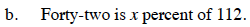$42=112\frac{x}{100}$

x = 37.5

See part (b).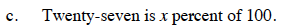0.27(500) = x